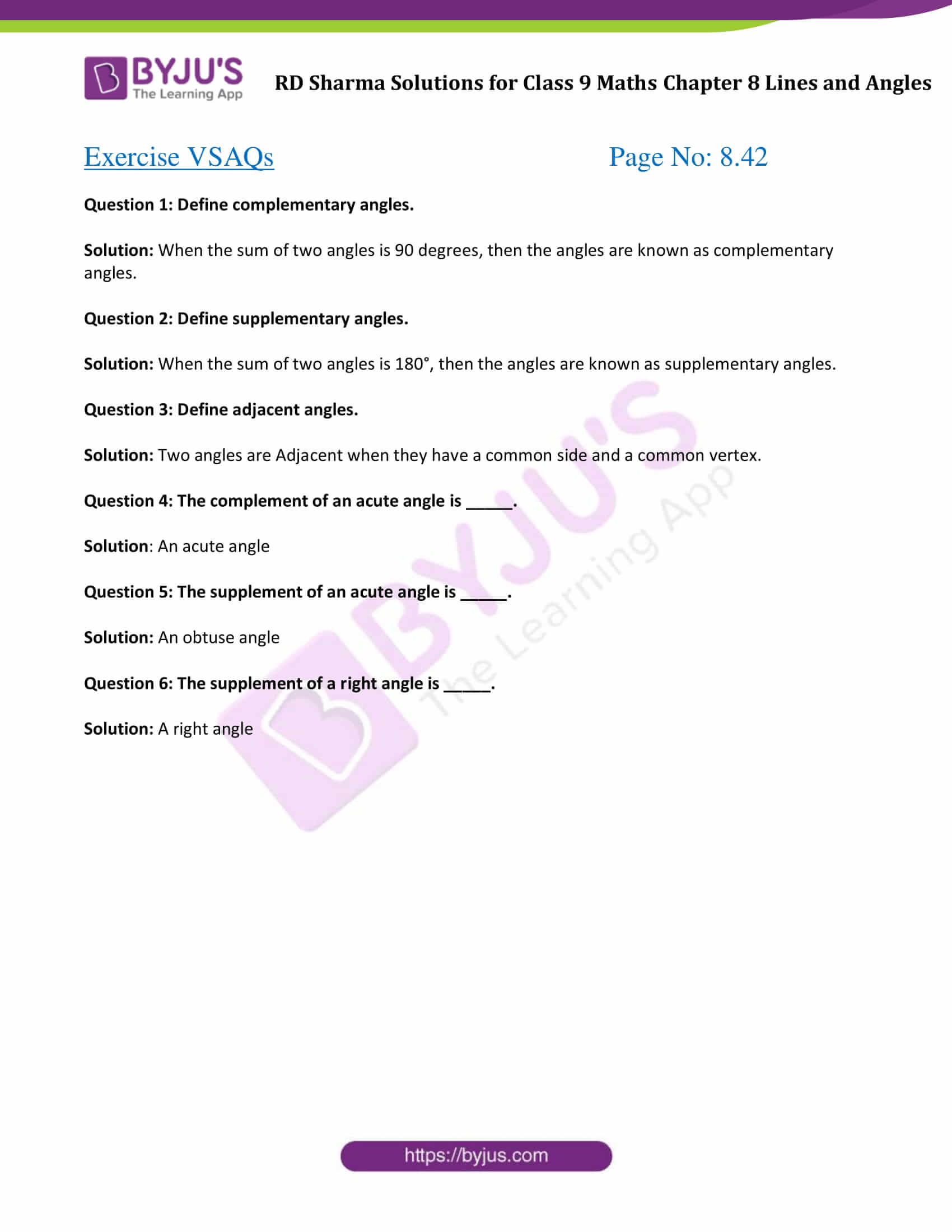# RD Sharma Solutions for Class 9 Maths Chapter 8 Lines and Angles Exercise VSAQs

RD Sharma Class 9 Maths solutions is provided here to help students master the concepts in chapter 8. This exercise questions are solved by subject experts at BYJU’S using a step-by-step problem-solving approach. This study material of RD Sharma Class 9 Solutions helps students to discover new ways to solve difficult problems.

## Download PDF of RD Sharma Solutions for Class 9 Maths Chapter 8 Lines and Angles Exercise VSAQs### Access Answers to Maths RD Sharma Solutions for Class 9 Chapter 8 Lines and Angles Exercise VSAQs Page number 8.42

Question 1: Define complementary angles.

Solution: When the sum of two angles is 90 degrees, then the angles are known as complementary angles.

Question 2: Define supplementary angles.

Solution: When the sum of two angles is 180°, then the angles are known as supplementary angles.

Solution: Two angles are Adjacent when they have a common side and a common vertex.

Question 4: The complement of an acute angle is _____.

Solution: An acute angle

Question 5: The supplement of an acute angle is _____.

Solution: An obtuse angle

Question 6: The supplement of a right angle is _____.

Solution: A right angle

## RD Sharma Solutions for Class 9 Maths Chapter 8 Lines and Angles Exercise VSAQs

Class 9 Maths Chapter 8 Lines and Angles Exercise VSAQs helps students to revise all the concepts covered under chapter 8. After practising RD Sharma Solutions chapter 8 Maths all exercise questions, students will be able to solve any question at their own pace.